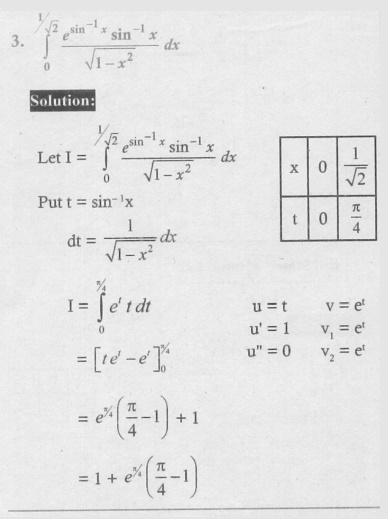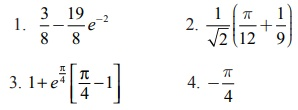Home | | Maths 12th Std | Exercise 9.4: BernoulliŌĆÖs Formula

# Exercise 9.4: BernoulliŌĆÖs Formula

Maths Book back answers and solution for Exercise questions - Mathematics : Applications of Integration : BernoulliŌĆÖs Formula - Exercise Problem Questions with Answer, Solution

EXERCISE 9.4

Evaluate the following: